# The second derivative, inflection point

## The concept of the second derivative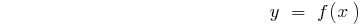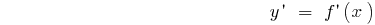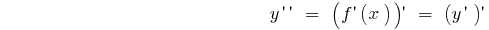Suppose the function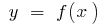has a derivative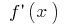at all points of some interval. This derivative, in turn, is a function of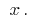If the functionis differentiated, its derivative is called the second derivativeand denoted(or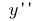)

Example.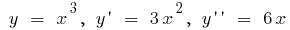## The concept of convexity, concavity and points of inflection of the graph funct

Let the functiondefined on the interval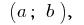and at the point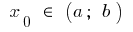has a finite derivative. Then schedule this function at the pointcan hold tangent

If in some neighborhood of the point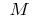all the points of the curve graph of a function(except for the points) lie above the tangent line, then we say that the curve (function) at the pointis convex (more precisely, strictly convex). Also it is sometimes said that in this case the function graphis convex down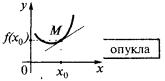If in some neighborhood of the pointall the points of the curve (except the points) lie below the tangent, then we say that the curve (function) at the pointis potou (or rather, strictly potou). Also it is sometimes said that in this case the function graph is convex upIf the point is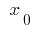on the x-axis has the property that if the argumentthrough the curvepasses from one side to the other tangent, then the pointis called the inflection point of the functionpoint curve— point of inflection of the graph of a function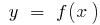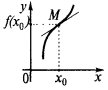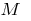the point of inflection of the graph of a function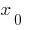the inflection point of the function

In some neighborhood of the point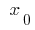: whenthe curve is below the tangent, and when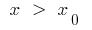the curve is above the tangent (or Vice versa)

## The study of the function of the bulge, unott and inflection points

Example.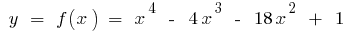1. To find the scope and the intervals on which the function is continuous
2. Scope: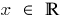The function is continuous at every point of its domain of definition

3. Find the second derivative
4.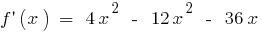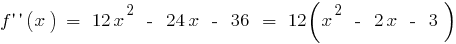5. Find an internal point of determining where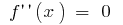or not there
6.there is in the entire scopewhen7. Mark the resulting point on the scope, find the sign of the second derivative and the behavior of the function on each interval, which splits the definition area
8.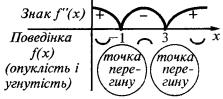9. To record the desired outcome of the study (intervals of convexity and concavity and points of inflection)
10. In the interval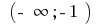and in the intervalgraph of a functionconvexity directed downwards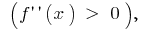and in the interval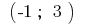the graph of the functionsent bump upInflection points:i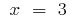(at these pointschanges the sign)

Tags:
Chapter:
Versions in other languages:
Share with friends: